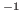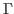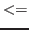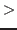Next: Test case Up: Description of the program Previous: Graphical representation of phonons

## Input variables and Input/Output files

A full list of input variables is reported in Table 1, and a list of the input/output files needed/generated by PHON is reported in Table 2

Table 1: Input variables
 Input variable Meaning Variable type Default NTYPES Number of atomic types Integer No default MASS Mass of atoms Real*NTYPES 1.0 LSUPER Generate supercell Logical .T. NDIM Supercell dimensions Integer*3 (1,1,1) LFREE Thermodynamic properties Logical .F. TEMPERATURE Temperature Real No default PTEMP Temperature increment, number of increments Real*2 (0.0, 1.0) LFORCEOUT Writes force constant matrix file Logical .F. LSYMM Symmetrises force constant matrix Logical .T. SYMPREC Precision threshold Real 1d-5 DXSTART Initial displacement Real*3 (1.0, 0.0, 0.0) DISP One over size of displacement (in Å) Integer 25 (0.04 Å) LCENTRAL For central differences Logical .F. (Forward differences) QA, QB, QC Number of divisions for q-points grid Integers No defaults LGAMMA q-points grid throughLogical .F. DOSIN, DOSEND, DOSSTEP Density of states parameters Reals 0.0, 25.0, 0.1 (THz) DOSSMEAR Width of Gaussian convoluted with DOS Real 0.02 (THz) USETHIS Partial density of states Logic*NTYPES .T. (total DOS) ND Number of q-points in dispersion segments Integer 0 QI, QF Initial and final points for dispersion segments Real*3 (0.0,0.0.0.0), (0.0,0.0,0.0) LRECIP q-points in reciprocal lattice coordinates Logical .T. NTI Imposes translational invariance Integer 1 (no TI imposed) IPRINT Controls verbosity of output Integer 0 (little output) LEIGEN Graphical representation of vibrations Logical .F. (no graphics) NAME? Name of atoms in the primitive cell Character*NTYPES H EIGSIZE Amplitude of vibrations Real 1.0 NCYCLESEIG Number of vibration cycles Integer 2

Table 2: Input/Output files
 File name Input/Output Description of data INPHON Input Input variables POSCAR Input Supercell FORCES Input Forces on all atoms (eV/Å), for each displacement QPOINTS Input/Output q-points for thermodynamics DISP Output Suggestions for displacements SPOSCAR Output File containing supercell FREQ, FREQ.cm Output (if natoms16) Phonon dispersions (THz and cm) FREQi, i=1,natoms/16 Output (if natoms16) Phonon dispersions in groups of 48 (THz) DOS, DOS.cm, DOS.meV Output Density of states (THz, cm, meV) THERMO Output Thermodynamic properties HARMONIC Output Force constant matrix EIGEN.axsf Output Eigenvectors (for representation with XCrySDen) MODE???.axsf Output Phonon vibrations (for representation with XCrySDen)Next: Test case Up: Description of the program Previous: Graphical representation of phonons
Dario Alfe` 2012-02-20